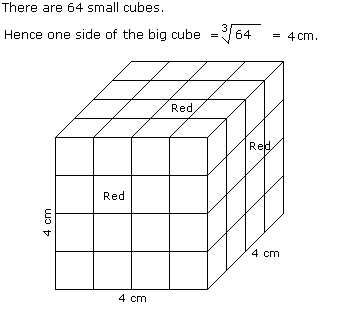# Verbal Reasoning - Cube and Cuboid - Discussion

The following questions are based on the information given below:

1. All the faces of cubes are painted with red colour.
2. The cubes is cut into 64 equal small cubes.

3.

How many small cubes are there whose three faces are coloured ?

 [A]. 4 [B]. 8 [C]. 16 [D]. 24

Explanation:Number of small cubes having three faces coloured = No. of corners = 8